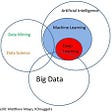# How to run a Machine Learning Gradient Boosting Regressor model for the Numerai Competition in under 5 mins

I’m at my best when I’m running through models — Future Hendrix

Hey all you cool cats and kittens, in this article I will be sharing my version of a simple numerai submission. Brief overview of what this entails. First, we’ll get the data straight from the source. Numerai has its own API so we can import this python library real quick. After getting the data, we import a machine learning library then use the regressor model. Predict the targets, evaluate and then submit it to Numerai.

First things first Rest In Peace Uncle Phil.

Btw, Numerai is a quantative hedge fund which hosts a data science tournament to crowdsource its data to trade on global markets and actually not invested into crypto, however to incentivize data scientist around the world particents in this tournament get rewarded in their crypto NMR. So far, Numerai reports its blowing the other quant hedge funds out the water and I’ve made over 2 months rent in NMR so I think this tournament is a perfect way to test my models for the market as well as learn earn some on side income.

# Observe

This numerai problem is a classification task giving us data with a target to predict the classification of a future target. I use regressor based on how numerai evaluates your models performance.

I will use google colab since its a 12gb of ram and you can share it with cohorts for the free. In colab many libraries are already installed so the only one will pip install will be the Numerai API.

`!pip install numerapiimport osimport gcimport csvimport scipyimport numerapiimport numpy as npimport pandas as pdimport matplotlib.pyplot as plt`

Below is the code downloads the data gets the latest round information including the training data and tournament data. Typically training and validation data don’t change every 3 or 4 weeks but luckily this model we run is very quick so we don’t need to worry if our model hasn’t changed from a previous round.

`def read_csv(filepath):  with open(filepath, 'r') as f:    column_names = next(csv.reader(f))    dtypes = {x: np.float16 for x in column_names if               x.startswith(('feature', 'target'))}  return pd.read_csv(filepath, dtype=dtypes)TARGET_NAME = f"target"PREDICTION_NAME = f"prediction"napi = numerapi.NumerAPI("Your", "Keys") # write your keys insteadnapi.download_current_dataset(unzip=True)currentr = napi.get_current_round()LatestRound = os.path.join('numerai_dataset_'+str(currentr))`

In machine learning when we train models with usually have a bunch of data and we split it into 2 parts. Training and testing are split 80% and 20% respectively. Numerai already has done it for us. Many data scientist will say ‘tHe ReAl wOrlD iSn’T liKE tHat’ but cleaning up data is a simple sometimes annoying task so if its already done lets take it as a W. Example preds are just an example of what your predictions should look like feel free to.

`training_data = read_csv(os.path.join(LatestRound, "numerai_training_data.csv")).set_index("id")tournament_data = read_csv(os.path.join(LatestRound, "numerai_tournament_data.csv")).set_index("id")example_preds = read_csv(os.path.join(LatestRound, "example_predictions.csv"))validation_data = tournament_data[tournament_data.data_type == "validation"]feature_names = [f for f in training_data.columns if f.startswith("feature")]cols = feature_names+['TARGET_NAME']`

I lied. We had to pip install catboost for our model. Catboost like any other python library is rather easy to use. If you want you can download anaconda and run it on your own computer so you can save the model and just pip install the packages just the one time. The .fit part takes the training features and targets. The eval_set takes the validation data aka test data and tunes its hyperparameters to create the model. Last week it took 22 secs so LFG.

`!pip install catboostfrom catboost import CatBoostRegressorModelfile = "damodel.cbm"params = {'task_type': 'GPU' }model = CatBoostRegressor(**params)model.fit(training_data[feature_names].astype(np.float64), training_data[TARGET_NAME].astype(np.float64),eval_set=(validation_data[feature_names].astype(np.float64), validation_data[TARGET_NAME].astype(np.float64)))model.save_model(Modelfile)`

To get some predictions, its pretty simple call .predict using the features you want. We get both so we can run some diagonstics.

`training_preds = model.predict(training_data[feature_names].astype(np.float64))training_data[PREDICTION_NAME] = training_predstournament_preds = model.predict(tournament_data[feature_names].astype(np.float64))tournament_data[PREDICTION_NAME] = tournament_press`

We use correaltion to get an idea of how well our model performed. We get 2 different correaltion scores one for the training set and one for the test set.

Unfornuately our model is overfit but works. Overfitting is when a model is overtrained and learned the training set too well but doesn’t perform as well out of sample like the test data.

Feature engineering is where we innovate and get better. Let this begining of your adventure data science journey.

`def correlation(predictions, targets):  ranked_preds = predictions.rank(pct=True, method="first")  return np.corrcoef(ranked_preds, targets)[0, 1]def score(df):  return correlation(df[PREDICTION_NAME], df[TARGET_NAME])# Check the per-era correlations on the training set (in sample)train_correlations = training_data.groupby("era").apply(score)validation_data = tournament_data[tournament_data.data_type == "validation"]validation_correlations = validation_data.groupby("era").apply(score)`

That’s it LFG. Submission time. Check out its performance in my link below.

`tournament_data[PREDICTION_NAME].to_csv("submission.csv", header=True)model_id = " YOUR MODEL ID"submission_id = napi.upload_predictions("submission.csv", model_id=model_id)`

ITS CHEWSDAY INNIT

https://numer.ai/dabeesknees48

--

--

--

## More from David Cruz

Tall Brown Math/ Graduate student in mathematics focusing in financial math

Love podcasts or audiobooks? Learn on the go with our new app.

## The Current State of the Art in Natural Language Generation (NLG)## BERT-based Cross-Lingual Question Answering with DeepPavlov## Hasty mistakes to be avoided by Novice Machine Learning Engineer## Sentiment Analysis, Part 1 — A friendly guide to Sentiment Analysis## Dog Breed Classifier using CNNs## [Notes] Improving Language Understanding by Generative Pre-Training## Remember Your Path to Success — Why Machine Learning has the potential to exceed human knowledge.## Machine Learning for Tax## David Cruz

Tall Brown Math/ Graduate student in mathematics focusing in financial math

## Testing the accuracy of your models predictions## Predicting Apple’s Stock Price Using A LSTM Recurrent Neural Network## ML Optimisation for Portfolio Allocation## Building Deep Learning Model to Predict Stock Prices (Part 1/2)